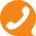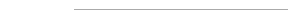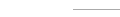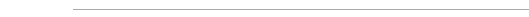### 东莞市德应东电子有限公司### 0769-8791883513312936462

0769-87918835

# 可调电位器怎么看阻值？两分钟带您学会101=100ohm 102=1000hm=1K

103=10000hm=10K 104=100000hm=100K

105=1000000ohm=1000K=1M 501=500ohm

502=5000hm=5K 503=50000hm=50K

504=500000hm=500K 505=5000000ohm=5000K=5M• 东莞市德应东电子有限公司
• 联系人：王先生
• 手机：13312936462
• 电话：0769-87918835
• 传真：0769-87916396
• 邮箱：wangweidong_168@126.com
• 网址：www.deyingdong.com
• 地址：东莞市塘厦镇石鼓村桥蛟中路122号5栋